# Chapter 21 Coulombs Law Copyright 2014 John Wiley

• Slides: 1721 -1 Coulomb’s Law Learning Objectives 21. 01 Distinguish between being electrically neutral, negatively charged, and positively charged and identify excess charge. 21. 04 Identify conduction electrons and explain their role in making a conducting object negatively or positively charged. 21. 02 Distinguish between conductors, nonconductors (insulators), semiconductors, and superconductors. 21. 05 Identify what is meant by “electrically isolated” and by “grounding. ” 21. 03 Describe the electrical properties of the particles inside an atom. 21. 06 Explain how a charged object can set up induced charge in a second object. 21. 07 Identify that charges with the same electrical sign repel each other and those with opposite electrical signs attract each other. © 2014 John Wiley & Sons, Inc. All rights reserved.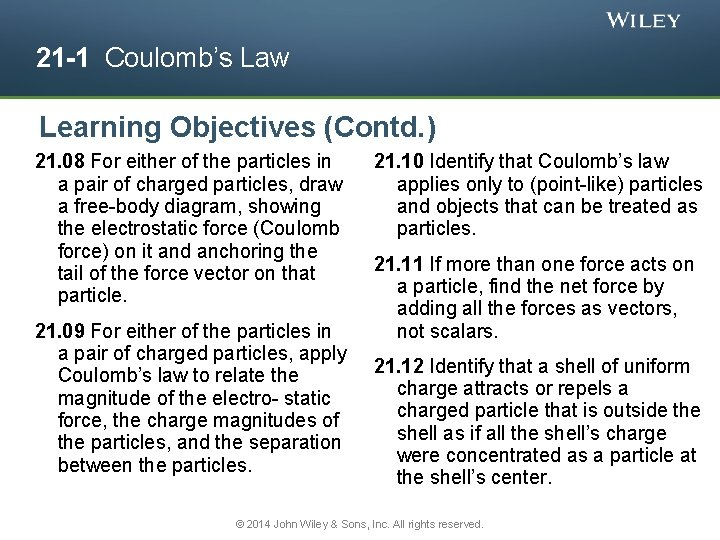21 -1 Coulomb’s Law Learning Objectives (Contd. ) 21. 08 For either of the particles in a pair of charged particles, draw a free-body diagram, showing the electrostatic force (Coulomb force) on it and anchoring the tail of the force vector on that particle. 21. 09 For either of the particles in a pair of charged particles, apply Coulomb’s law to relate the magnitude of the electro- static force, the charge magnitudes of the particles, and the separation between the particles. 21. 10 Identify that Coulomb’s law applies only to (point-like) particles and objects that can be treated as particles. 21. 11 If more than one force acts on a particle, find the net force by adding all the forces as vectors, not scalars. 21. 12 Identify that a shell of uniform charge attracts or repels a charged particle that is outside the shell as if all the shell’s charge were concentrated as a particle at the shell’s center. © 2014 John Wiley & Sons, Inc. All rights reserved.21 -1 Coulomb’s Law Learning Objectives (Contd. ) 21. 13 Identify that if a charged particle is located inside a shell of uniform charge, there is no net electrostatic force on the particle from the shell. 21. 14 Identify that if excess charge is put on a spherical conductor, it spreads out uniformly over the external surface area. 21. 15 Identify that if two identical spherical conductors touch or are connected by conducting wire, any excess charge will be shared equally. 21. 16 Identify that a non-conducting object can have any given distribution of charge, including charge at interior points. 21. 17 Identify current as the rate at which charge moves through a point. 21. 18 For current through a point, apply the relationship between the current, a time interval, and the amount of charge that moves through the point in that time interval. © 2014 John Wiley & Sons, Inc. All rights reserved.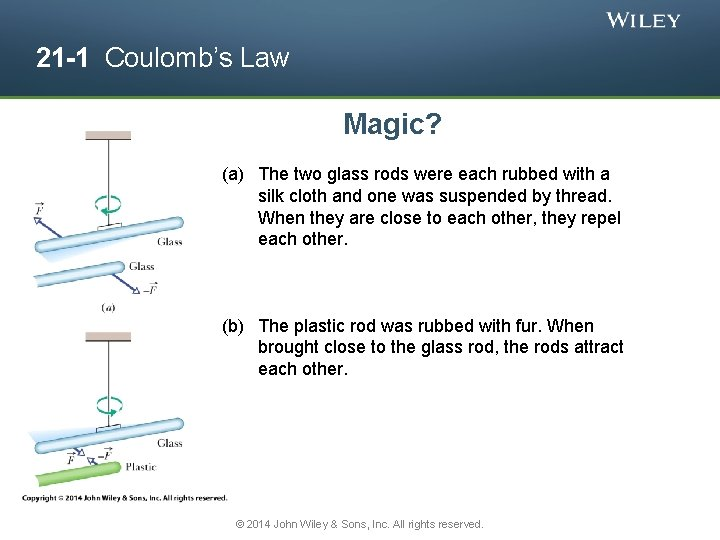21 -1 Coulomb’s Law Magic? (a) The two glass rods were each rubbed with a silk cloth and one was suspended by thread. When they are close to each other, they repel each other. (b) The plastic rod was rubbed with fur. When brought close to the glass rod, the rods attract each other. © 2014 John Wiley & Sons, Inc. All rights reserved.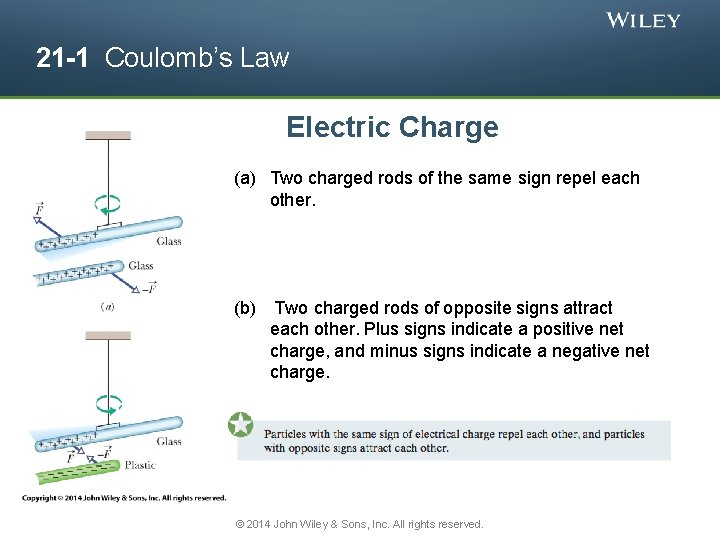21 -1 Coulomb’s Law Electric Charge (a) Two charged rods of the same sign repel each other. (b) Two charged rods of opposite signs attract each other. Plus signs indicate a positive net charge, and minus signs indicate a negative net charge. © 2014 John Wiley & Sons, Inc. All rights reserved.21 -1 Coulomb’s Law Materials classified based on their ability to move charge • Conductors are materials in which a significant number of electrons are free to move. Examples include metals. • The charged particles in nonconductors (insulators) are not free to move. Examples include rubber, plastic, glass. • Semiconductors are materials that are intermediate between conductors and insulators; examples include silicon and germanium in computer chips. • Superconductors are materials that are perfect conductors, allowing charge to move without any hindrance. © 2014 John Wiley & Sons, Inc. All rights reserved.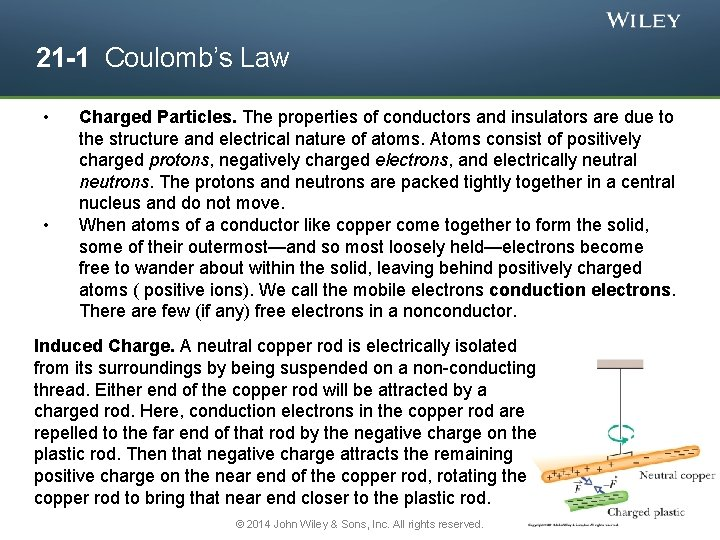21 -1 Coulomb’s Law • • Charged Particles. The properties of conductors and insulators are due to the structure and electrical nature of atoms. Atoms consist of positively charged protons, negatively charged electrons, and electrically neutral neutrons. The protons and neutrons are packed tightly together in a central nucleus and do not move. When atoms of a conductor like copper come together to form the solid, some of their outermost—and so most loosely held—electrons become free to wander about within the solid, leaving behind positively charged atoms ( positive ions). We call the mobile electrons conduction electrons. There are few (if any) free electrons in a nonconductor. Induced Charge. A neutral copper rod is electrically isolated from its surroundings by being suspended on a non-conducting thread. Either end of the copper rod will be attracted by a charged rod. Here, conduction electrons in the copper rod are repelled to the far end of that rod by the negative charge on the plastic rod. Then that negative charge attracts the remaining positive charge on the near end of the copper rod, rotating the copper rod to bring that near end closer to the plastic rod. © 2014 John Wiley & Sons, Inc. All rights reserved.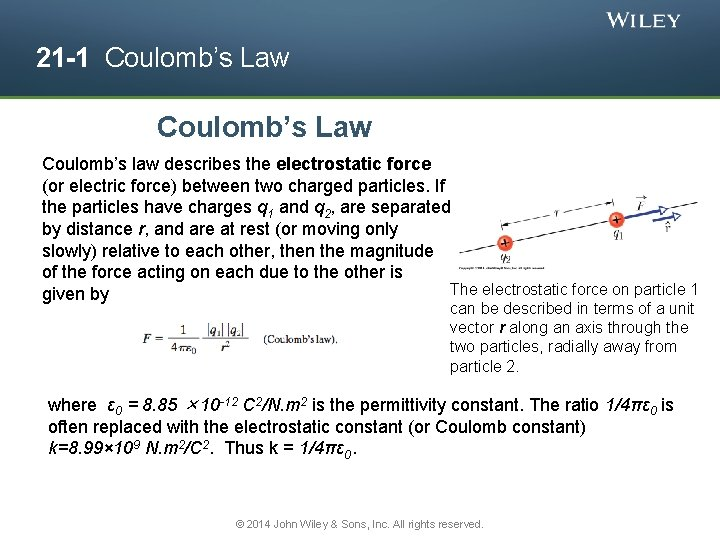21 -1 Coulomb’s Law Coulomb’s law describes the electrostatic force (or electric force) between two charged particles. If the particles have charges q 1 and q 2, are separated by distance r, and are at rest (or moving only slowly) relative to each other, then the magnitude of the force acting on each due to the other is The electrostatic force on particle 1 given by can be described in terms of a unit vector r along an axis through the two particles, radially away from particle 2. where ε 0 = 8. 85 × 10 -12 C 2/N. m 2 is the permittivity constant. The ratio 1/4πε 0 is often replaced with the electrostatic constant (or Coulomb constant) k=8. 99× 109 N. m 2/C 2. Thus k = 1/4πε 0. © 2014 John Wiley & Sons, Inc. All rights reserved.21 -1 Coulomb’s Law • The electrostatic force vector acting on a charged particle due to a second charged particle is either directly toward the second particle (opposite signs of charge) or directly away from it (same sign of charge). • If multiple electrostatic forces act on a particle, the net force is the vector sum (not scalar sum) of the individual forces. Two charged particles repel each other if they have the same sign of charge, either (a) both positive or (b) both negative. (c) They attract each other if they have opposite signs of charge. © 2014 John Wiley & Sons, Inc. All rights reserved.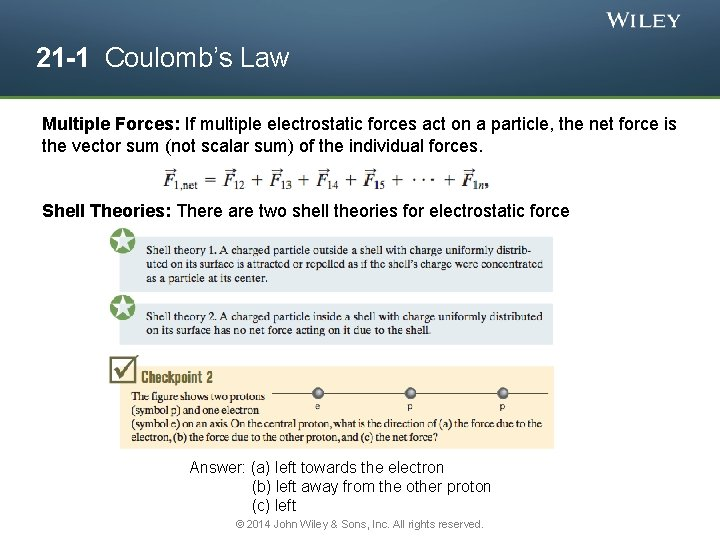21 -1 Coulomb’s Law Multiple Forces: If multiple electrostatic forces act on a particle, the net force is the vector sum (not scalar sum) of the individual forces. Shell Theories: There are two shell theories for electrostatic force Answer: (a) left towards the electron (b) left away from the other proton (c) left © 2014 John Wiley & Sons, Inc. All rights reserved.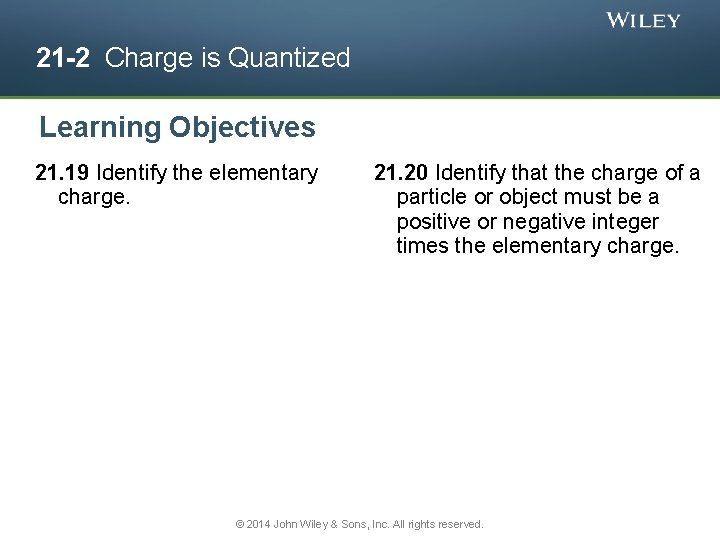21 -2 Charge is Quantized Learning Objectives 21. 19 Identify the elementary charge. 21. 20 Identify that the charge of a particle or object must be a positive or negative integer times the elementary charge. © 2014 John Wiley & Sons, Inc. All rights reserved.21 -2 Charge is Quantized • Electric charge is quantized (restricted to certain values). • The charge of a particle can be written as ne, where n is a positive or negative integer and e is the elementary charge. Any positive or negative charge q that can be detected can be written as in which e, the elementary charge, has the approximate value © 2014 John Wiley & Sons, Inc. All rights reserved.21 -2 Charge is Quantized When a physical quantity such as charge can have only discrete values rather than any value, we say that the quantity is quantized. It is possible, for example, to find a particle that has no charge at all or a charge of +10 e or -6 e, but not a particle with a charge of, say, 3. 57 e. Answer: -15 e © 2014 John Wiley & Sons, Inc. All rights reserved.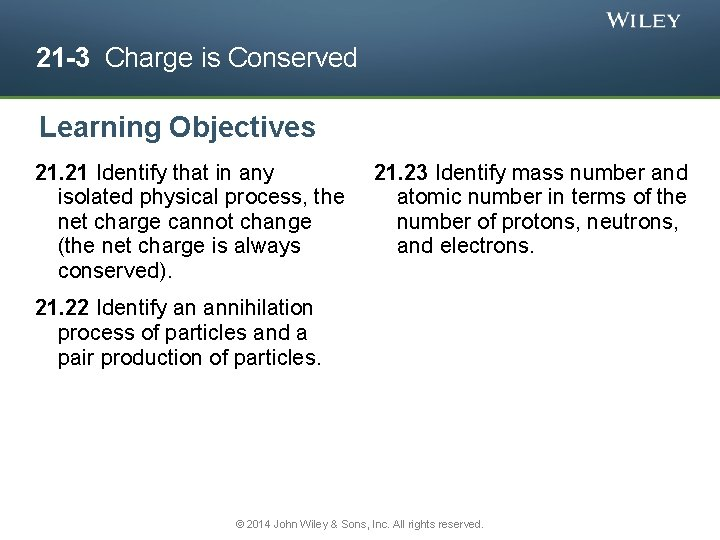21 -3 Charge is Conserved Learning Objectives 21. 21 Identify that in any isolated physical process, the net charge cannot change (the net charge is always conserved). 21. 23 Identify mass number and atomic number in terms of the number of protons, neutrons, and electrons. 21. 22 Identify an annihilation process of particles and a pair production of particles. © 2014 John Wiley & Sons, Inc. All rights reserved.21 -3 Charge is Conserved The net electric charge of any isolated system is always conserved. If two charged particles undergo an annihilation process, they have equal and opposite signs of charge. If two charged particles appear as a result of a pair production process, they have equal and opposite signs of charge. A photograph of trails of bubbles left in a bubble chamber by an electron and a positron. The pair of particles was produced by a gamma ray that entered the chamber directly from the bottom. Being electrically neutral, the gamma ray did not generate a telltale trail of bubbles along its path, as the electron and positron did. © 2014 John Wiley & Sons, Inc. All rights reserved.21 Summary Electric Charge Coulomb’s Law • The strength of a particle’s electrical interaction with objects around it depends on its electric charge, which can be either positive or negative. • The magnitude of the electrical force between two charged particles is proportional to the product of their charges and inversely proportional to the square of their separation distance. Conductors and Insulators • Conductors are materials in which a significant number of electrons are free to move. The charged particles in nonconductors (insulators) are not free to move. Conservation of Charge • . Eq. 21 -4 The Elementary Charge • Electric charge is quantized (restricted to certain values). • e is the elementary charge • The net electric charge of any isolated system is always conserved. © 2014 John Wiley & Sons, Inc. All rights reserved. Eq. 21 -12